# 1st PUC Physics Question Bank Chapter 3 Motion in a Straight Line

Students can Download Physics Chapter 3 Motion in a Straight Line Questions and Answers, Notes Pdf, 1st PUC Physics Question Bank with Answers helps you to revise the complete Karnataka State Board Syllabus and to clear all their doubts, score well in final exams.

## Karnataka 1st PUC Physics Question Bank Chapter 3 Motion in a Straight Line

Introduction

Question 1.
What is motion?
Motion is the change in position of an object with time.

Question 2.
What is uniform motion?
The object moving along the straight line covers equal distances in equal intervals of time is called uniform motion.

Question 3.
What is Mechanics?
Mechanics is the branch of physics that deals with the conditions of rest or motion of the objects around us.

Question 4.
What is Statics?
It is the branch of mechanics that deals with the study of objects at rest or in equilibrium.Question 5.
What is Dynamics?
It is the branch of Mechanics that deals with the study of motion of objects considering the cause of motion.

Question 6.
What is kinematics?
Kinematics deals with the study of motion of bodies without considering the causes of motion.

Position, Path Length and Displacement

Question 1.
What is required to specify the position of an object?
To specify the position of the object, a reference point called origin is required.

Question 2.
What is reference point or origin?
The point of intersection of three axes (x, y, z) is called origin and serves as the reference point.

Question 3.
What is meant by path length?
The total distance travelled by an object is called path length.

Question 4.
Define the term distance?
It is the length of the actual path travelled by a body between its initial and final positions.

Question 5.
What is displacement?
The change of position in a particular direction or the shortest distance between the initial and final position of the object is called displacement.
∆x = x2 – x1
Note: Displacement has both magnitude and direction (vector quantity).Question 6.
What are the characteristics of displacement?

1. Displacement has the same units of length.
2. Displacement is the shortest path that takes the object from its initial to the final position.

Question 7.
What is rectilinear motion?
The motion of an object along a straight line is known as rectilinear motion.

The Velocity Calculator is a handy physics tool to quickly get your answer.

Question 8.
What does the slope of position – time graph represent?
Velocity

Question 9.
Draw the position – time graph of (a) Stationary object (b) Uniform motion (c) A car in a motion starting from rest.Velocity, Speed and Acceleration

Question 1.
Define the term speed. Is it a scalar or vector? Give its S.I. unit.
The rate of change of position of an object without any reference to direction is called speed.
Speed = $$\frac{\text { Distance travelled by an object }}{\text { Time taken }}$$
It is a scalar quantity.
S.I. unit of speed is ms-1.Question 2.
Define uniform speed, and variable speed.
Uniform Speed:- An object is said to be moving with uniform speed, if it covers equal distances in equal intervals of time, however small the time interval may be.
Variable Speed:- An object is said to be moving with variable speed, if it covers unequal distances in equal intervals of time.

Question 3.
Define average speed.
Average speed is defined as the total path length travelled divided by the total time taken.
Average speed = $$\frac{\text { Path length }}{\text { Total time interval }}$$

Question 4.
Define instantaneous speed of a body.
The speed of an object at any particular instant of time or at a particular point of its path is called instantaneous speed of the object.
v = $$\lim _{\Delta x \rightarrow 0} \frac{\Delta x}{\Delta t}=\frac{d x}{d t}$$

Question 5.
Define the term velocity. Is it a scalar or a vector? Give its S.I. unit.
The rate of change of displacement of an object is called velocity.
It is a vector quantity
S.I. unit is ms-1.

Question 6.
Define uniform velocity and variable velocity.
Uniform velocity: A body is said to be moving with uniform velocity if it covers equal displacements in equal intervals of time, however small the time interval may be.

Variable velocity: A body is said to be moving with variable velocity if covers unequal displacements in equal in intervals of time.The Average Velocity calculator calculates average acceleration for entered values of initial, final velocity and time, Its, Calculate Average Velocity.

Question 7.
Define average velocity.
Average velocity is defined as the ratio of change in displacement (∆x) by time interval (∆t)
$$\bar{v}=\frac{x_{2}-x_{1}}{t_{2}-t_{1}}=\frac{\Delta x}{\Delta t}$$
Note: (i) S.I. Unit of velocity is ms-1
(ii) Average velocity can be positive velocity or negative velocity depending upon the sign of the displacement.

Question 8.
Distinguish between average speed and average velocity of a particle.
Average speed

1. The ratio of total distance travelled to the total time gives the average speed.
2. Average speed is a scalar physical quantity.
3. Average speed of a particle is finite; cannot be zero and always positive.
4. Average speed is greater than the magnitude of average velocity.

Average velocity

1. The ratio of net displacement of the particle to the total time taken gives the average velocity.
2. Average velocity is a vector physical quantity.
3. Average velocity of a particle may be zero, negative and positive.
4. Average velocity (magnitude) is less than the speed of the particle.

Question 9.
Define instantaneous velocity of a body in terms of its average velocity.
The instantaneous velocity is defined as the limit of the average velocity as the time interval At tends to zero.
v = $$\lim _{\Delta t \rightarrow 0} \frac{\Delta x}{\Delta t}=\frac{d x}{d t}$$
Velocity of an object (or) at a particular instant of time of its path (motion)

Question 10.
Define acceleration. Is it a scalar or a vector, Give its S J. unit.
The rate of change of velocity of an object with time is called acceleration.
It is a vector quantity. S.I. unit is ms-2.Question 11.
Define uniform acceleration and variable acceleration.
Uniform acceleration: The acceleration of an object is said to be uniform if its velocity changes by equal amount in equal intervals of time, however small the time interval may be.
Variable acceleration:- The acceleration of an object is said to be variable of its velocity changes by unequal amounts in equal intervals of time.

Question 12.
Define average acceleration.
Average acceleration is the ratio of change in velocity by the time interval during which the change occurs
a = $$\frac{\Delta v}{\Delta t}$$

Question 13.
Define instantaneous acceleration.
Acceleration of an object at a particular instant of time of its motion.
a = $$\lim _{A \rightarrow 0} \frac{\Delta v}{\Delta t}=\frac{d v}{d t}$$

Question 14.
Define retardation or deceleration? Give one example.
If the velocity of an object decreases with time, is called negative acceleration or deceleration or retardation. Example: On applying brakes on a moving car, the car comes to rest.

Question 15.
Draw the position – time graph for an object moving with (a) positive velocity (b) negative velocity (c) at rest.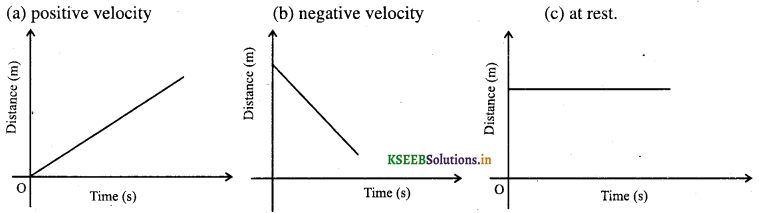Question 16.
Draw the position – time graph for motion with (a) positive acceleration (b) negative acceleration (c) zero acceleration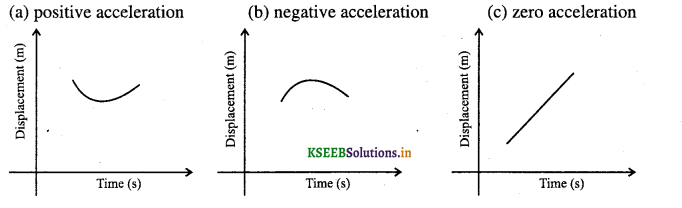Question 17.
What does area under velocity – time graph represent?
Displacement for a given time interval.

Question 18.
What does the slope of velocity – time graph represent?
Acceleration

Question 19.
What is the acceleration of a body moving with constant velocity?
Zero.Question 20.
Draw the velocity time – graph for motion in (a) Positive direction with positive acceleration (b) Positive direction with negative acceleration (c) Negative direction with negative acceleration.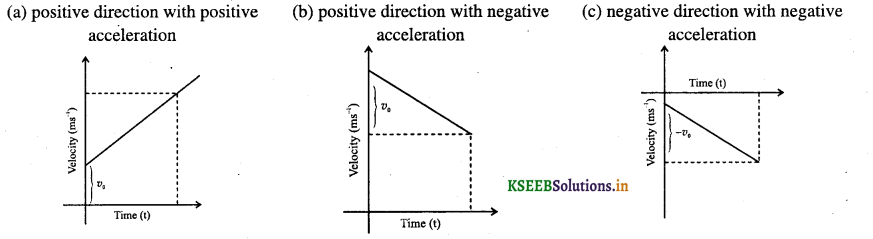Note: The area under the curve (dotted line) represents the displacement over given interval of time.

Question 21.
What are the uses of velocity-time graph?
From the velocity-time graph,

1. the nature of motion of a particle can be determined.
2. velocity and hence distance of a particle can be predicted well in advance for a uniform acceleration.
3. the area bounded by the velocity-time graph determines the distance travelled by the particle.
4. the instantaneous velocity of a particle, distance covered during the nlh second and acceleration in terms of distances covered during consecutive equal intervals of time can be calculated.

Kinetic Equations for Uniformly Accelerated Motion

Question 1.
Derive the equation of motion x = v0t + $$\frac{1}{2}$$ at2 from v-t graph.
Consider an object moving with an initial velocity Vo under constant acceleration ‘a’. After ‘t’ second, let v be its velocity and x the displacement. Let AB represent the velocity – time curve of the object. Here OA represent v0, DB represents v and OD represents Y.
The area under v-t curve represents the displacement
∴ x = area under AB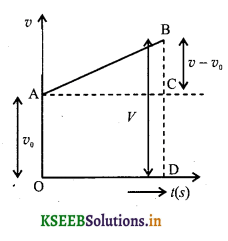= area of the rectangle OACD + area of ∆ABC
= (OA X OD ) + $$\frac{1}{2}$$ (AC X BC)
= V0t + $$\frac{1}{2}$$t(v – v0 ) ……. (1)
But v = v0 = at
∴ v – v0 = at
substituting this in equation (1) we get
x = v0t + $$\frac{1}{2}$$at2

Question 2.
Derive the equation of motion for v = v0 + at.
Consider an object moving with an initial velocity VQ under constant acceleration ‘a’. After Y seconds, V be its velocity and V the displacement. Let AB represent the velocity – time curve. Here OA represents v0, DB represents V and OD represent Y.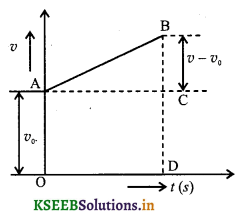The slope of velocity – time graph represents uniform acceleration ‘a’
∴  Accelaration = slope = $$\frac{B C}{A C}$$
∵ AC = OD = t
∴   a = $$\frac{v-v_{0}}{t}$$
v – v0 = at
v = v0 + at …… (1)

Question 3.
Derive the equations of motion for v2 = v02 + lax from v-t graph.
Consider an object moving with initial velocity Vo under constant acceleration a’. After Y second, V be its velocity and x the displacement. Let AB represent the velocity – time graph. Here OA represents v0, OB represents V and OD represents ‘t’
The slope of velocity – time graph represents uniform accelaration ‘a’
∴ accelaration = slope = $$\frac{B C}{A C}$$
∵  AC = OD = t
a = $$\frac{v-v_{0}}{t}$$
v – v0 = at
v = v0 + at …… (1)
The object has traveled distance ‘x’ in time ‘t’ with average velocity V given asWe can obtain the most general equation if we take the position coordinate at t = 0 as non zero say x0. The above equation can be modified by replacing x by x – x0
v2 = v02 + 2a{x – x0)Question 4.
Obtain equations of motion for constant acceleration using method of calculus.
By definition
a = $$\frac{d v}{d t}$$
Integrating both sides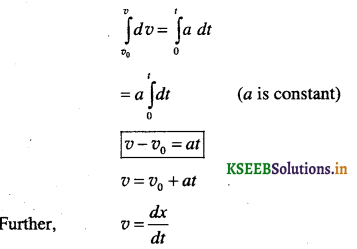dx = vdt
Integrating both sides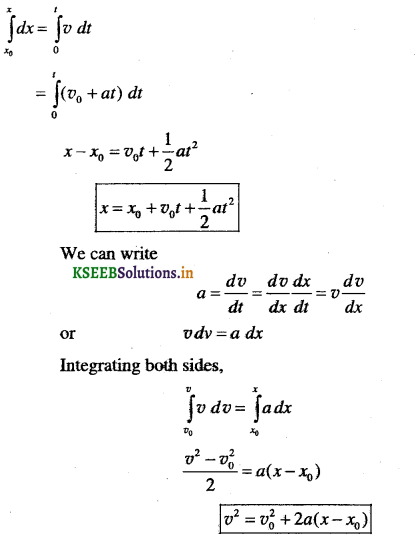Relative Velocity

Question 1.
Define Relative velocity.
The velocity of an object wife respect to another object when both are in motion is called relative velocity.

Question 2.
Deduce an expression for relative velocity of one object with respect to another.
Consider two objects A and B moving along x – axis uniformly with average velocities vA and vB respectively.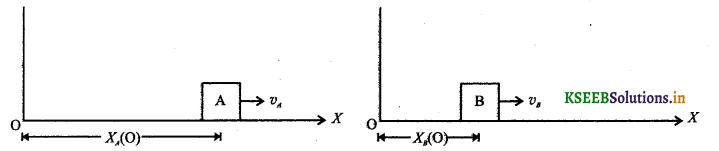Let xA(0) and xB(0) be their displacement from the origin O at t = 0
Let xA(t) and xB(t) be their displacement at time ‘t’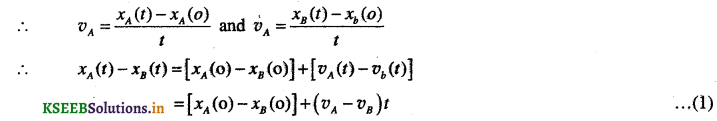Change in displacement between the two bodies in time t
= [xA(t) – xB(t) ] – [xA(o) – xB(o)]
= [xA(o) – xB(o) ] – (vA – vB)t] – [xA(o) – [xB(o) ]because of eq. (1)
= (vA – vB)t
therefore relative velocity of A w.r.t B is
VAB = $$\frac{\text { Change in displacement }}{\text { time }}=\frac{\left(v_{A}-v_{B}\right) t}{t}$$
∴  VAB = VA – VB
Change in displacement _ (vA – vB)t
Similarly, we can show that relative velocity of B w.r.t A is VBA = VB – VAQuestion 3.
Two objects A and B are moving with average velocity v each. What is the their relative velocity if they are moving in (a) same direction (b) opposite director.
(a) v – v = 0
(b) v – (-v) = 2v

Question 4.
Draw position-time graphs for two objects moving along a straight line, when their relative velocity is
(a) Zero, (b) Positive and (c) Negative.
When v1 = v2Question 5.
When does the magnitude of displacement is equal to the total path length?
When the motion of an object is along a straight line and in the same direction the magnitude of displacement is equal to the total path length.

Question 6.
Generally average sped is not equal to the magnitude of the average velocity. Give reason.
Answer:This is because the motion involves change in direction so that the path length is greater than the magnitude of displacement. This shows that speed is greater than the magnitude of the velocity.

Question 7.
How do you determine the instantaneous velocity position – time graph?
By taking slope of the tangent to the graph at that instant.

Question 8.
When does velocity is same as the average velocity at all instants?
In uniform motion.

Question 9.
Express instantaneous acceleration of a particle for a non-uniform accelerated motion mathematically.
Acceleration v = $$\lim _{\Delta x \rightarrow 0} \frac{\Delta v}{\Delta t}=\frac{d v}{d t}$$

Numerical Problems

Question 10.
A car is moving along a straight line, say OP in figure. It moves from O to P in 18 s and returns from
P to Q in 6.0 s. What are the average velocity and average speed of the car in going (a) from O to P? and
(b) from O to P and back to Q?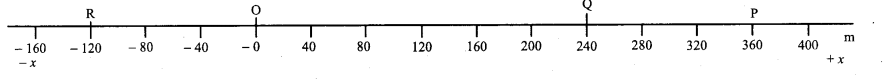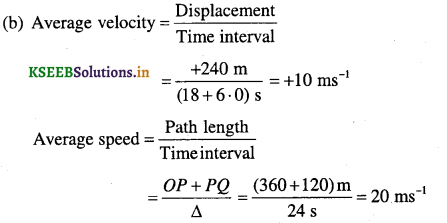Question 11.
A car moving along a straight highway with speed of 126 km h’ 1 is brought to a stop within a distance of 200 m. What is the retardation of the car (assumed uniform), and how long does it take for the car to stop?
V0 = 126km h-1 = 126 x $$\frac{5}{18}$$ ms-1, v = 0
Stopping distance = (x – x0) = 200 m
Since the car is stopped, v = 0.
v2 = v02 + 2 a(x – x0)
0 = 352 + 2a(200)
a = -3.06 ms-2
Retardation = 3.06 ms-2
v = v0 + at
0 = 35 – 3.06t
= 11.4 s
Time for car to stop = 11.4 s.

Question 12.
A ball is thrown upwards with a speed of 100 ms-1. Show that the all hits the ground with the same speed after returning from the highest point. Neglect air resistances.
For upwards journey of the ball u = 10 ms-1, v = 0 a = -g
Let A be the maximum distance travelled by the ball in the upwards direction.
v2 = u2 +2gh
v2 – u2 = 2gh .
02 – (100)2 =-2 x 10 x h
h = 500 m
For downward journey of the ball
u = 0, h = -500 m, V = ?
v2 – u2 = 2gh
v2 =10,000
∴  v = 100 ms-1Question 13.
Two trains A and B of length 400 m each are moving on two parallel tracks with a uniform speed of 72 km h1 in the same direction, with A ahead of B. The driver of B decides to overtake A and accelerates by 1 ms’2. If after 50 s, the guard of B Just brushes past the driver of A, what was the original distance between them?
Initially, relative acceleration = a = 1 ms-2
v0 = 72 km h-1 = 72 x $$\frac{5}{18}$$ ms-1 = 20 ms-1
relative displacement = x
relative velocity initially = 0
x = $$\frac{1}{2}$$ at2
= $$\frac{1}{2}$$ x 1 x 502 = 1250 m
Original distance between the trains = 1250 m.Question 14.
On a two – lane road, car A is travelling with a speed of 36 km h-1. Two cars B and C approach car A in opposite directions with a speed of 54 km h-1 each. At a certain instant, when the distance AB is equal to AC, both being 1 km, B decides to overtake A before C does. What minimum acceleration of car B is required to avoid an accident?
vA = 36 km h-1= 10 ms-1, vB = vC = 54 km h-1 = 15 ms-1
Velocity of B with respect to A, vBA = vB – vA
= 15 m – 10 = 5 ms-1
Velocity of C with respect to A, vCA = vC – vA
= 15m -10 = 5 ms-1
Velocity of C with respect to A, vCA = vC – vA
= 15 – (- 10) = 25 ms-1
s = VCAt
1000 = 25 x t
t = 40 s
The car C will take 40 s to cover AC.
In that time, B must cover AB to overtake and avoid collisions.
s = vBAt +$$\frac{1}{2}$$at2
1000 = 5 x 40 + $$\frac{1}{2}$$ x a x 402
⇒ a = 1 ms-2
Acceleration of B = 1 ms-2Question 15.
A jet airplane travelling at the speed of 500 km h-1 ejects its products of combustion at the speed of 1500 km h-1 relative to the jet plane. What is the speed of the latter with respect to an observer on the ground?
Speed of the exhaust with respect to an observer on the ground = Speed of exhaust with respect to the plane –
Speed of plane with respect to the ground.
(Negative sign because the plane and exhaust are opposite directions)
= (1500 – 500) km h-1 = 1000 km h-1

Question 16.
Two towns A and B are connected by a regular bus service with a bus leaving in either direction every T minutes. A man cycling with a speed of 20 km h-1 in the direction A to B notices that a bus goes past him every 18 min in the direction of his motion, and every 6 min in the opposite direction. What is the period T at the bus service and with what speed (assumed constant) do the buses ply on the road?
Let the speed of the bus be v km h-1
Distance covered by one bus before the next one leaves the same town = vT
For buses going in the same direction as the cyclist, frequency of the bus is 18 min i.e.,Question 17.
A player throws a ball upwards with an initial speed of 29.4 ms
(a) What is the direction of acceleration during the upward motion of the ball?
(b) What are the velocity and acceleration of the ball at the highest point of its motion?
(c) Choose the x = 0 m and t = 0s to be the location and time of the ball at its highest point, vertically downward direction to be the positive direction of x-axis, and give the signs of position, velocity and acceleration of the ball during Its upward, and downward motion.
(d) To what height does the ball rise and after how long does the ball return to the player’s hands?
(Take g = 9.8 m s-2 and neglect air resistance). .
(a) The direction of acceleration is vertically downwards (towards the Earth)

(b) At the highest point, velocity = 0 ms-1 acceleration = g = 9.8 m s-2 vertically – downwards.

(c) During upward motion:
Position : positive
Velocity : negative
Acceleration : positive
During downward motion:
Position : positive
Velocity : positive
Acceleration : positive

(d) v = 0 at the highest point, v0 = 29.4 ms-1, g = -9.8 ms-2
v2 = v02 + 2 gh
0 = 29-42 = 2 X (-9-8) X 4
h = 44.1 m
Height of ascension = 44.1 m
V = V0 + g t
0 = 29.4 – 9.8 t
t = 3s
Time of ascent = Time of descent
∴ Time for the ball to return 6 s.Question 18.
Read each statement below carefully and state with reasons and examples. If it Is true or false;
A particle in one-dimensional motion
(a) with zero speed at an instant may have non-zero acceleration at that instant
(b) with zero speed may have non-zero velocity,
(c) with constant speed must have zero acceleration,
(d) with positive value of acceleration must be speeding up.